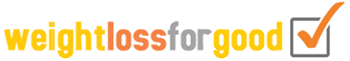# Height Conversion

When you need to change feet to centimetres, our height conversion tool makes things really easy.

Likewise if you need to change metric to imperial for whatever reason, you can use the same height converter calculator below to get the correct values.

Once you have the right figures, then why not use for the BMI Calculator, the Body Fat Calculator, and the Calorie Calculator.

If you need to convert weight units for the above calculators, use the Weight Conversion page.

## The Height Conversion calculator

 Enter figure : Convert from: centimetres feet inches meters To: centimetres feet inches meters Result